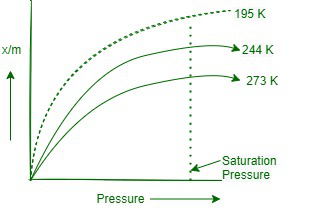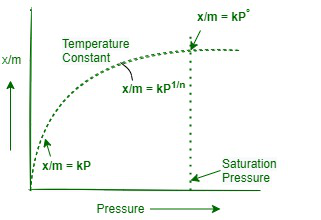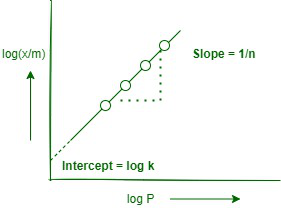GFG App
Open AppBrowser
Continue

The phenomenon of the congregation of the highest concentration of any molecular species on a surface than the agglomeration of a solid (or liquid) is called adsorption. It deals with the chemistry at the range which separates two bulk phases. This range is also known as surface and interface. △H and △S of adsorption are negative. Most adsorption processes are exothermic, therefore, adsorption decreases with increasing temperature.

1. The adsorbent is an extract on the surface that has adsorption on its surface.
2. Adsorbate is the type that concentrates or accumulates on the surface.
3. In adsorption, the concentration of the adsorbent increases only on the surface of the adsorbent, whereas in absorption, the concentration is uniform throughout the bulk of the solid. Adsorption is a surface phenomenon whereas absorption is a bulk phenomenon, e.g. water vapours are absorbed by anhydrous CaCl, but adsorbed by silica gel.
4. The term sorption is used when both the processes of adsorption and adsorption occur simultaneously.
5. Desorption is the process of removing an adsorbed substance from the surface on which it is adsorbed.

Factors Affecting Adsorption of Gases on Solids

• Nature of adsorbent Greater are the strained forces on the surface, more is the case with which adsorption takes place on the surface. The activated adsorbents have high adsorbing power.
• The surface area of the adsorbent Greater the surface area, the more is the adsorption.
• Nature of gas being adsorbed Easily liquefiable gases like NH3, HCl, Cl2, SO2, CO2 etc. (whose critical temperature is high) is adsorbed to a greater extent.
• Pressure Adsorption increases with an increase in pressure. The effect of pressure is large at low temperatures.
• Temperature Since, adsorption is an exothermic process so according to Le-Chatelier’s principle adsorption decreases with an increase in temperature.

The amount of adsorption of a gas per unit mass of the adsorbent depends on the pressure of the gas. The relationship between the amount of adsorbent at a constant temperature and the pressure (or concentration for a solution) of the equilibrium gas is called the adsorption isotherm

It can be expressed as an equation or a graphical curve (or plot). The amount of adsorption is usually expressed as x/m where x is the mass of the adsorbent and m is the mass of the adsorbent. The simplest type of adsorption isIt is clear from the figure that the adsorption limit (x/m) increases as the pressure increases and reaches a maximum corresponding to the pressure P, which is called the equilibrium pressure. Since adsorption is a reversible process, adsorption also does not occur simultaneously. At this pressure (P), the volume of the adsorbed gas becomes equal to the volume of the dehydrated gas, so that the extent of the adsorbent remains constant even when the pressure is increased. This state is called saturation state and P is called saturation pressure. Scientists have tried to explain adsorption in terms of some empirical mathematical relationship called adsorption isotherm.

The variation of extent of adsorption (x/m) with pressure (P) at a particular temperature was given mathematically by Freundlich in 1909. From the adsorption isotherm as drawn, the following observations can be easily made as:

• At low pressure: The graph is an almost straight line which indicates that m is directly proportional to pressure. This may be expressed as-

x/m ∝ P

or

x/m = kP                                                                                                                                                                   … (i)

where k is a constant.

• At high pressure: The graph becomes almost constant which means that becomes independent of pressure. This may be expressed as-Adsorption isotherm in terms of Freundlich

x/m ∝ constant

or

x/m ∝ P° (P° = 1)                                                                                                                                                    … (ii)

x/m = kP°

• Thus, in the intermediate range of pressure, x/m will depend upon the power of pressure which is between 0 to 1 i.e., fractional power of range 0.1 to 0.5). This may be expressed as pressure (probable range 0.1 to 0.5). This may be expressed as:

x/m ∝ P1/n

x/m = kP1/n                                                                                                                                                         … (iii)

where n can take any whole number value which depends upon the nature of adsorbate and adsorbent. The above relationship is also called Freundlich’s adsorption isotherm.

• Calculation of k and n of adsorption isotherm: The constants and n can be determined as explained below:

Taking logarithms on both sides of Eq. (iii), we get

log(x/m)= log k + (1/n) log P                                                                                                                            … (iv)

Thus, if we plot a graph between log (x/m) on y (ordinate) and log P, on the x-axis (abscissa), a straight line will be obtained. This also shows the validity of Freundlich’s isotherm. The slope of the line is equal to Un and the intercept is equal to log k.Linear Graph between log(x/m) and log P

Limitations of Freundlich adsorption isotherm: Freundlich’s adsorption isotherm fails at the high pressure of the gas. Therefore, this relation is considered to approximate and is applicable only at low pressures. Irving Langmuir derived a simple adsorption isotherm on the theoretical considerations based on the kinetic theory of gases. This is named Langmuir adsorption isotherm.

### Sample Questions

Question 1. Distinguish between the meanings of the words adsorption and absorption. Give one example each.

Adsorption is a phenomenon in which the concentration of another substance is greater on the surface than in the bulk. Absorption is a phenomenon in which the molecules of one substance are evenly distributed throughout the body of another substance. For example, silica gel absorbs water vapor while anhydrous calcium chloride absorbs water.

Question 2. Give cause why nicely divided material is frequent emphatic as an adsorbent.

Finely divided materials have more surface area and hence more adsorption.

Question 3. Why are lyophilic colloidal sols much static than lyophobic colloidal sols?

Lyophilic colloidal sols are much static because they are highly hydrated in solution

Question 4. Give one test to distinguish whether the given emulsion is oil in water type or water in oil type emulsion.

It can be identified by dilution test. Thus, the emulsion is diluted with water. If the emulsion is diluted with water, it means that water serves as the dispersion medium and is an example of an oil in water emulsion. If, it is not dilute, the oil serves as the dispersion medium and this oil is an example of water in emulsion.

Question 5. What is observed when sodium chloride is added to a colloidal solution of ferric hydroxide?

When sodium chloride is added to ferric hydroxide sol, the sol. accumulates. This is because Clions from NaCl neutralizes the positive charge on Fe(OH) sol.

Question 6. What is the difference between sol and gel?